Estimating the mean value using R2jags

Estimating the mean value using R2jags

This file was generated on 2013-03-06 18:32:36

``````library(plyr)
library(reshape2)
library(ggplot2)
library(gridExtra)
library(R2jags)
library(mcmcplots)
library(xtable)
``````

Make data

Make some simulated data to analyze. This way, we know the true mean and standard errrors. Here, we make two sets of data with different number of samples. In the small set, we have 6 samples from a normal distribution with a mean of 600 and a standard error of 30. In the big set, we have 1000 samples from the same normal distribution.
``````smallset = rnorm(6, mean = 600, sd = 30)
bigset = rnorm(1000, mean = 600, sd = 30)
``````

GLM

Histogram plots
``````p1 = ggplot() + geom_histogram(aes(x = smallset)) + labs(x = "Small set of 10 samples")
p2 = ggplot() + geom_histogram(aes(x = bigset)) + labs(x = "Big set of 1000 samples")
grid.arrange(p1, p2)
``````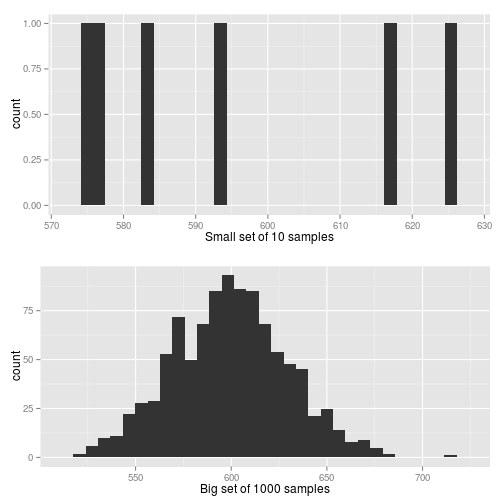The GLM for the small dataset
``````m1 = glm(smallset ~ 1, family = gaussian)
summary(m1)
``````
``````
Call:
glm(formula = smallset ~ 1, family = gaussian)

Deviance Residuals:
1      2      3      4      5      6
-19.9   30.6  -12.7  -18.7   22.0   -1.3

Coefficients:
Estimate Std. Error t value Pr(>|t|)
(Intercept)   595.57       8.81    67.6  1.3e-08 ***
---
Signif. codes:  0 '***' 0.001 '**' 0.01 '*' 0.05 '.' 0.1 ' ' 1

(Dispersion parameter for gaussian family taken to be 465.7)

Null deviance: 2328.3  on 5  degrees of freedom
Residual deviance: 2328.3  on 5  degrees of freedom
AIC: 56.79

Number of Fisher Scoring iterations: 2
``````
The GLM for the big dataset
``````m2 = glm(bigset ~ 1, family = gaussian)
summary(m2)
``````
``````
Call:
glm(formula = bigset ~ 1, family = gaussian)

Deviance Residuals:
Min      1Q  Median      3Q     Max
-76.85  -21.67    0.34   19.81  117.18

Coefficients:
Estimate Std. Error t value Pr(>|t|)
(Intercept)  599.438      0.952     630   <2e-16 ***
---
Signif. codes:  0 '***' 0.001 '**' 0.01 '*' 0.05 '.' 0.1 ' ' 1

(Dispersion parameter for gaussian family taken to be 905.9)

Null deviance: 905020  on 999  degrees of freedom
Residual deviance: 905020  on 999  degrees of freedom
AIC: 9650

Number of Fisher Scoring iterations: 2
``````

Jags model

Write the Jags model as a function. The order of the statements are irrelevant for Jags.
``````jagsmodel = function() {
# Priors
population.mean ~ dunif(0, 5000)
precision <- 1/population.variance
population.variance <- population.sd * population.sd
population.sd ~ dunif(0, 100)
# Likelihood
for (i in 1:nobs) {
obs[i] ~ dnorm(population.mean, precision)
}
}
``````
Set up the small data set for Jags.
``````obs = smallset
nobs = length(smallset)
jagsdata = list("obs", "nobs")
``````
Choose the parameters to monitor, this must be a character vector.
``````parameters = c("population.mean", "population.sd")
``````
Set up the initialization function for Jags.
``````inits = function() {
list(population.mean = runif(1, 0, 5000), population.sd = runif(1, 0, 100))
}
``````
We can run Jags in serial or in parallel.
``````# This is the code for serial execution.  jout <- jags(data=jagsdata,
# inits=inits, parameters.to.save=parameters, model.file=jagsmodel,
# n.chains=4, n.inter = 2*10^3, n.burnin = 10^3, n.thin = 5)

# This is the code for parallel execution.
jout.small <- jags.parallel(data = jagsdata, inits = inits, parameters.to.save = parameters,
model.file = jagsmodel, n.chains = 4, n.iter = 2 * 10^3, n.burnin = 10^3,
n.thin = 1)
``````
JAGS was run for 4 chains, 2000 iterations per chain, with a burnin of 1000 and thinning rate of 1.
When Jags is run in parallel, the print method doesn't seem to work for the jags object. However, we can access the names lists within it.
To get the summarized parameters.
``````jout.small\$BUGSoutput\$summary
``````
``````                  mean     sd   2.5%    25%    50%    75%  97.5%  Rhat
deviance         55.69  2.765  52.86  53.67  54.80  56.90  62.95 1.003
population.mean 595.89 13.123 568.43 588.39 595.58 602.92 624.58 1.002
population.sd    29.34 12.757  14.53  20.65  25.97  34.07  66.03 1.002
n.eff
deviance         1600
population.mean  2500
population.sd    1800
``````
Using the coda library makes examining the output much easier.
``````jout.small.mcmc = as.mcmc(jout.small)
``````
Gelman-Rubin Diagnostics and Plot
``````gelman.diag(jout.small.mcmc)
``````
``````Potential scale reduction factors:

Point est. Upper C.I.
deviance                 1       1.01
population.mean          1       1.01
population.sd            1       1.01

Multivariate psrf

1
``````
``````gelman.plot(jout.small.mcmc)
``````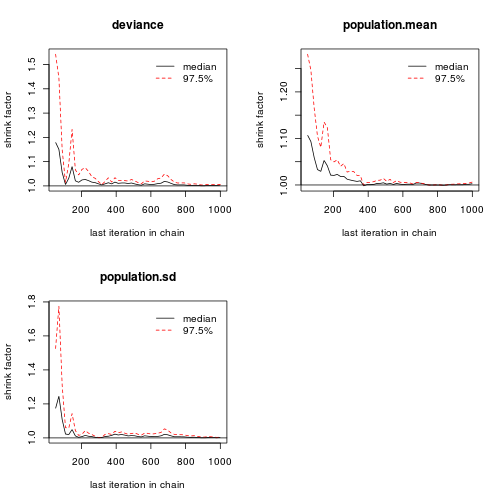Trace plots
``````traceplot(jout.small.mcmc)
``````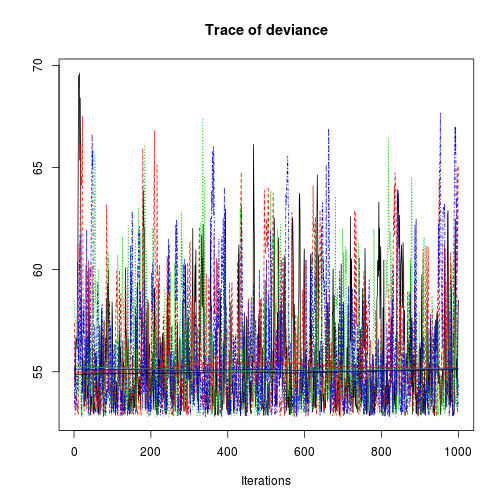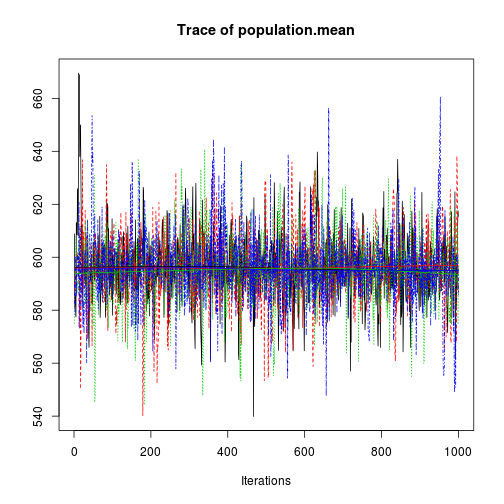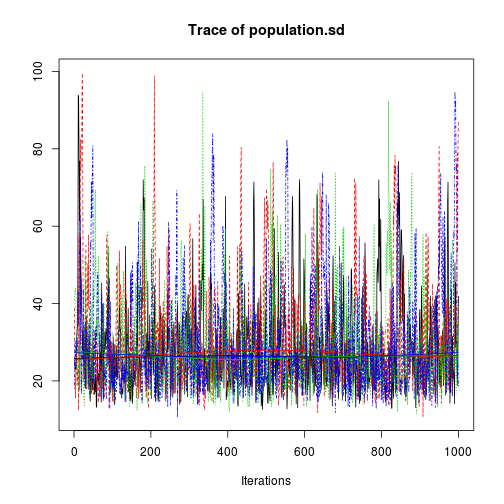Stack the lists in the mcmc object so that we can make a histogram of the posterior distributions.
``````jout.small.stack = rbind.fill.matrix(jout.small.mcmc)
``````
Take a look at the stacked matrix
``````head(jout.small.stack)
``````
``````     deviance population.mean population.sd
[1,]    55.06           608.9         23.91
[2,]    55.28           581.5         25.36
[3,]    54.27           585.6         24.27
[4,]    55.02           582.4         22.85
[5,]    56.71           577.4         23.59
[6,]    56.44           613.0         23.50
``````
Histogram of the population mean
``````qplot(x = jout.small.stack[, "population.mean"], geom = "histogram", xlab = "Popualation mean")
``````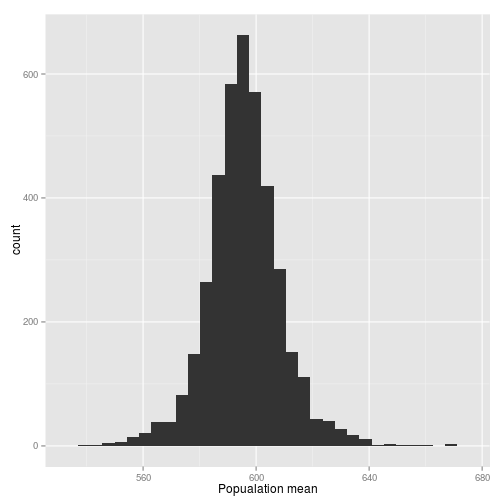Histogram of the population standard error
``````qplot(x = jout.small.stack[, "population.sd"], geom = "histogram", xlab = "Popualation mean")
``````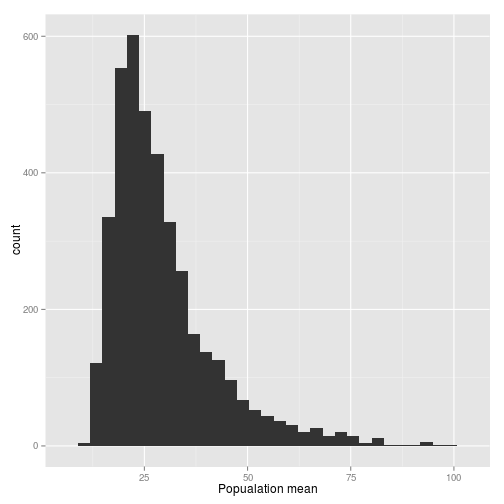Compare these with the true mean and standard error

Estimated values Standard error True values
population.mean 595.89 13.12 600.00
population.sd 29.34 12.76 30.00

If you increase the sample size, then the estimates should get better. Notice how the estimate for the standard error for the big set is close to the true standard error. Also, note that Jags provides the standard errors for the estimated parameters, including the standard error of the estimated SE parameter, whereas GLM does not provide a standard error for the estimate of SE The true mean is 600 and the true standard error is 30.

Mean values Standard error
Bayesian small set mean 595.9 13.1
Bayesian small set SE 29.3 12.8
Bayesian big set mean 599.4 1.0
Bayesian big set SE 30.1 0.7
GLM small set mean 595.6 8.8
GLM small set SE 21.6
GLM big set mean 599.4 1.0
GLM big set SE 30.1

This file took 0.11 minutes to generate.

Adapted from the workshop “Hierarchical Bayesian modeling for ecologists”, hosted by Y. Yamaura (Hokkaido University) and presented by M. Kéry (Swiss Ornithological Institute) at Sapporo in 2013.
-->